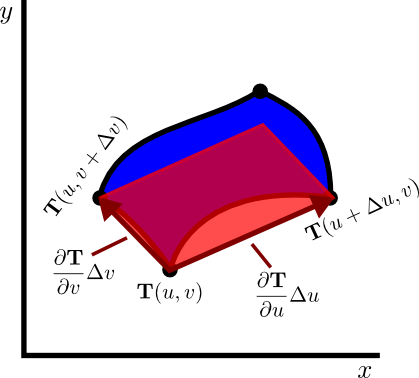# Math Insight

### Image: Approximating region as parallelogram to calculate area under transformationThe change of variables function $\cvarf$ transforms a small rectangle in the $\cvarfv\cvarsv$ plane to a “curvy rectangle” in the $xy$ plane. We approximate the area of the “curvy rectangle” by using a parallelogram.

Image file: change_variable_area_parallelogram.png

Source image file: change_variable_area_parallelogram.svg
Source image type: Inkscape SVG